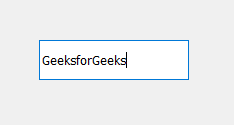Open in App
Not now

# PyQt5 – QLineEdit

• Last Updated : 18 Aug, 2020

QLineEdit : It allows the user to enter and edit a single line of plain text with a useful collection of editing functions, including undo and redo, cut and paste, and drag and drop. It is the basic widget in PyQt5 to receive keyboard input, input can be text, numbers or even symbol as well. Below is how the line edit look likeExample :
We will create a line edit and a label and when user enter text in it and when enter key is pressed, the label will display the entered text.

Below is the implementation

 `# importing libraries ` `from` `PyQt5.QtWidgets ``import` `*`  `from` `PyQt5 ``import` `QtCore, QtGui ` `from` `PyQt5.QtGui ``import` `*`  `from` `PyQt5.QtCore ``import` `*`  `import` `sys ` ` `  ` `  `class` `Window(QMainWindow): ` ` `  `    ``def` `__init__(``self``): ` `        ``super``().__init__() ` ` `  `        ``# setting title ` `        ``self``.setWindowTitle(``"Python "``) ` ` `  `        ``# setting geometry ` `        ``self``.setGeometry(``100``, ``100``, ``500``, ``400``) ` ` `  `        ``# calling method ` `        ``self``.UiComponents() ` ` `  `        ``# showing all the widgets ` `        ``self``.show() ` ` `  ` `  ` `  `    ``# method for components ` `    ``def` `UiComponents(``self``): ` ` `  `        ``# creating a QLineEdit object ` `        ``line_edit ``=` `QLineEdit(``"GeeksforGeeks"``, ``self``) ` ` `  `        ``# setting geometry ` `        ``line_edit.setGeometry(``80``, ``80``, ``150``, ``40``) ` ` `  `        ``# creating a label ` `        ``label ``=` `QLabel(``"GfG"``, ``self``) ` ` `  `        ``# setting geometry to the label ` `        ``label.setGeometry(``80``, ``150``, ``120``, ``60``) ` ` `  `        ``# setting word wrap property of label ` `        ``label.setWordWrap(``True``) ` ` `  `        ``# adding action to the line edit when enter key is pressed ` `        ``line_edit.returnPressed.connect(``lambda``: do_action()) ` ` `  `        ``# method to do action ` `        ``def` `do_action(): ` ` `  `            ``# getting text from the line edit ` `            ``value ``=` `line_edit.text() ` ` `  `            ``# setting text to the label ` `            ``label.setText(value) ` ` `  ` `  ` `  ` `  `# create pyqt5 app ` `App ``=` `QApplication(sys.argv) ` ` `  `# create the instance of our Window ` `window ``=` `Window() ` ` `  `# start the app ` `sys.exit(App.``exec``()) `

My Personal Notes arrow_drop_up
Related Articles